# DAV Class 8 Maths Chapter 10 Worksheet 3 Solutions

The DAV Maths Class 8 Solutions and DAV Class 8 Maths Chapter 10 Worksheet 3 Solutions of Parallel Lines offer comprehensive answers to textbook questions.

## DAV Class 8 Maths Ch 10 WS 3 Solutions

Question 1.
Draw a line segment AB = 5 cm and divide it internally into six equal parts.
Steps of Construction:
1. Draw a line segment AB = 5 cm.
2. Draw two parallel rays AP and BQ at A and B respectively making an acute angle.
3. Using compass mark equal distance A1, A2, A3, A4, A5 and A6 at AP and B1, B2, B3, B4, B5 and B6 at BQ.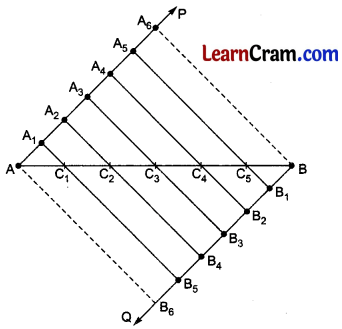4. Join AB6, A1B5, A2B4, A3B3, A4B2, A5B1 and A6B which cut AB at C1, C2, C3, C4, C5 respectively.
5. Measure $$\overline{\mathrm{AC}_1}, \overline{\mathrm{C}_1 \mathrm{C}_2}, \overline{\mathrm{C}_2 \mathrm{C}_3}, \overline{\mathrm{C}_3 \mathrm{C}_4}, \overline{\mathrm{C}_4 \mathrm{C}_5}$$ and C5B which are equal.

Question 2.
Draw a line segment of length 6.4 cm and divide it into four equal parts. What is the length of each part?
Steps of Construction:
1. Draw AB = 6.4 cm.
2. Draw rays $$\overrightarrow{\mathrm{AP}} \| \overrightarrow{\mathrm{BQ}}$$ at A and B respectively making acute angles with AB .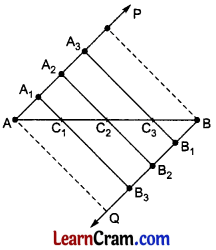3. Using compass, mark A1, A2, A3, and B1, B2, B3 on  and  at equal distances.
4. Join AQ, A1B3, A2B2, A3B1 and PB which cut AB at C1, C2 and C3.
5. Measure $$\overline{\mathrm{AC}_1}, \overline{\mathrm{C}_1 \mathrm{C}_2}, \overline{\mathrm{C}_2 \mathrm{C}_3}$$, C3B which are same and length of each part = 1.6 cm.Question 3.
Draw a line segment of length 5.5 cm. Divide it internally in the ratio 2 : 3. What is the length of each part?
Steps of Construction:
1. Draw $$\overline{\mathrm{AB}}$$ = 5.5 cm.
2. Draw $$\overrightarrow{\mathrm{AP}} \| \overrightarrow{\mathrm{BQ}}$$ at A and B respectively making acute angles.3. Mark A1, A2, A3, A4 and B1, B2, B3 and B4 on $$\overrightarrow{\mathrm{AP}}$$ and $$\overrightarrow{\mathrm{BP}}$$ respectively at equal distance.
4. Join AQ and BP.
5. Join A2B3 which cuts AB at C.
6. C is the required point which divides $$\overrightarrow{\mathrm{AB}}$$ in the ratio 2:3.

Question 4.
Draw a line segment of length 6.3 cm and divide it internally in the ratio 3 : 4.
Steps of Construction:
1. Draw $$\overline{\mathrm{AB}}$$ = 6.3 cm.
2. Draw $$\overrightarrow{\mathrm{AP}} \| \overrightarrow{\mathrm{BQ}}$$ at A and B respectively making acute angles with $$\overline{\mathrm{AB}}$$ in opposite sides.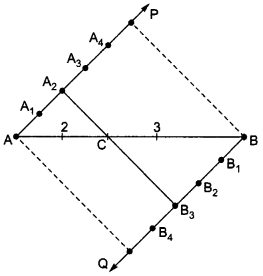3. Mark A1, A2, A3, A4, A5, A6 and B1, B2, B3, B4, B5, B6 on $$\overrightarrow{\mathrm{AP}}$$ and $$\overrightarrow{\mathrm{BQ}}$$ at equal distances.
4. Join AQ, BP and A3B4.
5. A3B4 cuts $$\overline{\mathrm{AB}}$$ at C which divides $$\overline{\mathrm{AB}}$$ in the ratio 3 : 4.

Question 5.
Draw a line segment AB of length 7 cm and find a point P on it such that $$\overline{\mathrm{AP}}: \overline{\mathrm{PB}}$$ =1:3. Measure $$\overline{\mathrm{AP}}$$ and $$\overline{\mathrm{PB}}$$.
Steps of Construction:
1. Draw $$\overline{\mathrm{AB}}$$ = 7 cm.
2. Draw $$\overline{\mathrm{AP}}: \overline{\mathrm{PB}}$$ at A and B respectively making acute angles on the opposite sides of AB.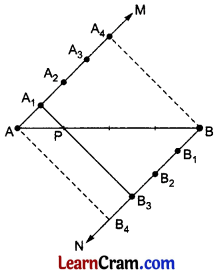3. With the help of compass, mark 1 + 3 = 4 points namely A1, A2, A3, A4 on $$\overrightarrow{\mathrm{AM}}$$ and B1; B2, B3, B4 on $$\overrightarrow{\mathrm{AM}}$$ at equal distances.
4. Join AB4 and BA4.
5. Join A1 to B3 which cuts AB at P to divides $$\overrightarrow{\mathrm{AB}}$$ in the ratio 1 : 3 i.e. $$\overline{\mathrm{AP}}: \overline{\mathrm{PB}}$$ =1:3.

Question 6.
Draw a line segment of a given length. Divide it into four equal parts.
Steps of Construction:
1. Draw $$\overline{\mathrm{AB}}$$ of any length.
2. Draw $$\overrightarrow{\mathrm{AP}} \| \overrightarrow{\mathrm{BP}}$$ at A and B respectively making acute angles on opposite sides of AB.3. With the help of compass, mark A1, A2 A3 and A4 on $$\overrightarrow{\mathrm{AP}}$$ and B1, B2, B3 and B4 on $$\overrightarrow{\mathrm{BQ}}$$ at equal distances.
4. Join AB4, A1B3, A3B2, A3B1 and A4B which cut $$\overline{\mathrm{AB}}$$ at C1, C2 and C3.
5. Hence, required divisions are
$$\overline{\mathrm{AC}_1}=\overline{\mathrm{C}_1 \mathrm{C}_2}=\overline{\mathrm{C}_2 \mathrm{C}_3}=\overline{\mathrm{C}_3 \mathrm{~B}}$$.Question 7.
Draw a line segment AB = 5.5 cm. Find a point P on it such that $$\overline{\mathrm{AP}}=\frac{2}{3} \overline{\mathrm{BP}}$$.
Steps of Construction:
1. Draw $$\overline{\mathrm{AB}}$$ = 5.5 cm.
2. Draw $$\overrightarrow{\mathrm{AM}} \| \overrightarrow{\mathrm{BN}}$$ at A and B respectively making acute angles on the opposite sides of $$\overline{\mathrm{AP}}=3 \overline{\mathrm{BP}}$$ .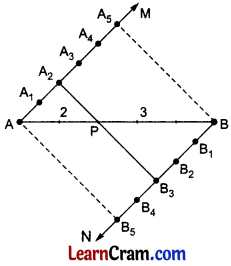3. With the help of compass, mark A1, A2 A3, A4, A5 on  and B1, B2, B3, B4, B5 on  at equal distances.
4. Join AB5 and BA5.
5. Join A2 to B3 which meets $$\overline{\mathrm{AB}}$$ at P such that $$\overline{\mathrm{AP}}: \overline{\mathrm{BP}}$$ = 2 : 3 or $$\overline{\mathrm{AP}}=3 \overline{\mathrm{BP}}$$.

Question 8.
Draw a line segment AB = 6 cm. Find a point Q on it such that $$\overline{\mathrm{AQ}}=\frac{2}{3} \overline{\mathrm{QB}}$$.
1. Draw $$\overline{\mathrm{AB}}$$ = 6 cm.
2. Draw $$\overrightarrow{\mathrm{AM}} \| \overrightarrow{\mathrm{BN}}$$ at A and B respectively making acute angles on the opposite sides of AB.
3. Mark A1; A2, A3, A4, A5 on $$\overrightarrow{\mathrm{AM}}$$ and B1; B2, B3, B4 and B5 on $$\overrightarrow{\mathrm{BN}}$$ at equal distances.5. Join A2B3 which cuts $$\overline{\mathrm{AB}}$$ at Q such that $$\overline{\mathrm{AQ}}: \overline{\mathrm{QB}}$$ = 2 : 3 i.e. $$\overline{\mathrm{AQ}}=\frac{2}{3} \overline{\mathrm{QB}}$$.